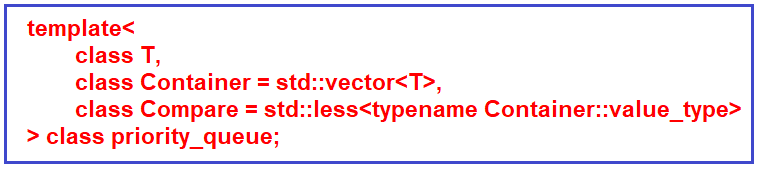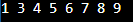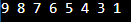# Priority Queue in C++

## Priority Queue in C++ with Examples:

In this article, I am going to discuss Priority Queue in C++ with Examples. Please read our previous article, where we discussed Queue in C++ with Examples.

##### Priority Queue in C++ with Examples:

Now, we will study another important container adapter that is called the priority queue. It provides a constant time lookup of the largest element (by default). It’s a queue where elements have different priorities. You will pick the element with the highest priority and by default, the highest priority will be given to the largest element.

By largest element means, we have a way of comparing the elements. For example, if we have integers 8 and 4 then we would say that 8 is more than 4. So, by default 8 will have higher priority. Although you can define your own priority. You can provide a custom compare function where you will define the order you want. Maybe in your use case, 4 has a higher priority so you can assign that. Insertions and extractions will take logarithmic time.

You need to include a ‘queue’ header file and a user-provided compare can be supplied to change the ordering. This comparison will be used for determining the priority and this will be defined as,So, you have a type of data ‘T’ that is stored in the container. It is an adapter so there is an underlying container and by default, the container will be a vector of the same type. Then you can provide the compare and if you don’t provide anything the default will be std::less. So, it will arrange the larger values ahead of a smaller values. So larger values will get higher priority although we will see an example of this also where we will provide our own custom comparator and we will change the priority.

class Compare = std::less<typename Container::value_type>

The above line will become mandatory when the element is of type some custom user-defined class and then you will need to define a custom comparator but even for primitive types you can define your own comparator. You can see here, one of the elements is the container and the default is a vector. Now let us see the container

##### Container in C++:

It is the type of underlying container to use to store the elements and the requirement is that whatever container you decide to use it has to meet the requirements of a sequence container. So, by sequence container, we mean a container that stores the object of the same type in a linear arrangement, and the iterators must satisfy the requirements of LegacyRandomAccessIterator which is a legacy bidirectional iterator that can be moved to point at any element in constant time. So random access is possible. And for example, a pointer to an element of the array satisfies the requirement of a legacy random access iterator. So, it has to satisfy this whatever container it is and in fact, vector satisfies that and it’s the default.

The queue also satisfies that requirement and another requirement was that it must provide the following functions: front() push_back(), and pop_back(). These must be there and these are supported by vector and deque.

Next, we said that we can define our own comparator, and if we don’t define it, the default will be std::less and it will arrange in descending order that is, 8 will be given higher priority than 4, 5, 3, 6, 2. If we change it to std::greater<T>, it will be reversed. So, we will see an example of that also.

##### Compare in C++:

It is a compare type that is providing strict weak ordering. Compare parameter is defined such that, it returns true if the first argument comes before its second argument in weak ordering. Let’s see some of the functions then we will jump to examples.

##### Priority Queue Functions in C++:
1. size() – it will return the number of elements in the underlying container.
2. = – equal is used to assign values to the container adapter.
3. top() – top accesses the top element that is the element with the highest priority depending on how you Have defined the priority and this will be of O (1) which is constant time.
4. empty() – it will check whether it’s empty or not if the number of elements in the underlying container is 0 then it is empty.
5. push() – It inserts the element and sorts the underlying container.
6. pop() – it removes the top element.

So, let us start with a simple example. We will see the default container and default comparator and then we will start modifying.

##### Example to Understand Default Container & Comparator in C++:
```#include <iostream>
#include <queue>
#include <vector>

using namespace std;

int main()
{
// Create a priority queue.
priority_queue<int, vector<int>, std::greater<int>> q;

// Create a vector to hold integers values.
vector<int> v = {3, 5, 8, 6, 7, 1, 9, 4};

// insert all the elements of vector v in the queue q.
for(int x : v) q.push(x);

while(!q.empty()){
cout << q.top() << " ";
q.pop();
}
cout << endl;

return 0;
}
```
###### Output:##### Example to understand How to Define our own Container & Comparator:
```#include <iostream>
#include <queue>
#include <vector>
using namespace std;

int main()
{
// Define our own comparator
auto cmp = [] (int a, int b){
return a < b;
};

// Create a priority queue.
priority_queue<int, vector<int>, decltype(cmp)> q(cmp);

// Create a vector to hold integers values.
vector<int> v = {3, 5, 8, 6, 7, 1, 9, 4};

// insert all the elements of vector v in the queue q.
for(int x : v) q.push(x);

// print the elements of queue and then remove them.
while(!q.empty()){
cout << q.top() << " ";
q.pop();
}
cout << endl;

return 0;
}
```
###### Output: